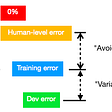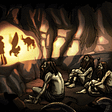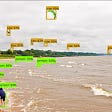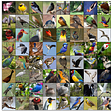# Face Recognition using Transfer Learning

• Problem Statement: Create a project using transfer learning solving various problems like Face Recognition, Image Classification, using existing Deep Learning models like VGG16, VGG19, ResNet, etc.

## What is Transfer Learning ?

• In ML , Transfer Learning is just a technique in which the knowledge gained by a model while training with a dataset is used for training it with some other dataset and here the knowledge is the weights calculated by the model.

In ML world , there are multiple pre-trained models available like VGG , MobileNet , ResNet , Inception etc. And in this project i have used MobileNet.

• So let’s get started with the project.
1. First i prepared dataset using cv2. I ran this code twice for collecting images of two persons.This is the code.I have used harcascade for detecting the face part so that i can crop the face part automatically.
`import cv2import numpy as np face_classifier = cv2.CascadeClassifier('haarcascade_frontalface_default.xml')# Function to detect face and give cropped face as outputdef face_extractor(img):        faces = face_classifier.detectMultiScale(img, 1.3, 5)        if faces is ():        return None    for (x,y,w,h) in faces:        cropped_face = img[y:y+h, x:x+w]    return cropped_face# Capturing frames using webcame <0 for default, 1 for external>cap = cv2.VideoCapture(0)count = 0while True:    ret, frame = cap.read()    if face_extractor(frame) is not None:        count += 1        face = cv2.resize(face_extractor(frame), (200, 200))                # Save file in specified directory with unique name        file_name_path = 'C://Users//91721//Desktop//ml_task4//images//image' + str(count) + '.jpg'        cv2.imwrite(file_name_path, face)        # Put count on images and display live count        cv2.putText(face, str(count), (50, 50), cv2.FONT_HERSHEY_COMPLEX, 1, (0,0,255), 3)        cv2.imshow('Face Cropper', face)            else:        print("Error finding face")        pass    if cv2.waitKey(1) == 13 or count == 100:        break        cap.release()cv2.destroyAllWindows()print("Data collected !!")`
• In this i have collected 100 images for training and 20 images for validation but this much images are not enough to train the model as it will not able to recognize the features of our face precisely and also we get less accuracy. So for increasing our dataset we can use Augmentation which basically rotate and flip our images to create new images and by this we can create thousands of images from just 100 images.
• Now we have to load the MobileNet model and for this we will download the weights of this model from Imagenet and since we have to do transfer learning and don’t have to train the whole model again , so we will freeze all the layers of the model and then create 4 more new layers and train these layers only.
• Now after all this we have to load our dataset and then train the model.
`from keras.models import Sequentialfrom keras.layers import Dense, Dropout, Activation, Flatten, GlobalAveragePooling2Dfrom keras.layers import Conv2D, MaxPooling2D, ZeroPadding2Dfrom keras.layers.normalization import BatchNormalizationfrom keras.models import Modelfrom keras.optimizers import RMSpropfrom keras.callbacks import ModelCheckpoint, EarlyStoppingfrom keras.preprocessing.image import ImageDataGenerator# Load MobileNet modelfrom keras.applications import MobileNet# MobileNet was designed to work on 224 x 224 pixel input images sizesimg_rows, img_cols = 224, 224# Re-loads the MobileNet model without the top or FC layersMobileNet = MobileNet(weights = 'imagenet',                  include_top = False,                  input_shape = (img_rows, img_cols, 3))# freezing the last 4 layers # Layers are set to trainable as True by defaultfor layer in MobileNet.layers:    layer.trainable = False # function that returns FC Headdef lw(bottom_model, num_classes):    """creates the top or head of the model that will be     placed ontop of the bottom layers"""top_model = bottom_model.output    top_model = GlobalAveragePooling2D()(top_model)    top_model = Dense(1024,activation='relu')(top_model)    top_model = Dense(1024,activation='relu')(top_model)    top_model = Dense(512,activation='relu')(top_model)    top_model = Dense(num_classes,activation='softmax')(top_model)    return top_model    num_classes = 2FC_Head = lw(MobileNet, num_classes)model = Model(inputs = MobileNet.input, outputs = FC_Head)# Loading Datasettrain_data_dir = 'C://Users//91721//Desktop//ml_task4//'validation_data_dir = 'C://Users//91721//Desktop//ml_task4//'# Data augmentaiton train_datagen = ImageDataGenerator(      rescale=1./255,      rotation_range=45,      width_shift_range=0.3,      height_shift_range=0.3,      horizontal_flip=True,      fill_mode='nearest') validation_datagen = ImageDataGenerator(rescale=1./255) # setting the batch size (typically on most mid tier systems we'll use 16-32)batch_size = 32 train_generator = train_datagen.flow_from_directory(        train_data_dir,        target_size=(img_rows, img_cols),        batch_size=batch_size,        class_mode='categorical') validation_generator = validation_datagen.flow_from_directory(        validation_data_dir,        target_size=(img_rows, img_cols),        batch_size=batch_size,        class_mode='categorical')        checkpoint = ModelCheckpoint("task3/myface_model.h5",                             monitor="val_loss",                             mode="min",                             save_best_only = True,                             verbose=1)earlystop = EarlyStopping(monitor = 'val_loss',                           min_delta = 0,                           patience = 3,                          verbose = 1,                          restore_best_weights = True)# putting call backs into a callback listcallbacks = [earlystop, checkpoint]# using a very small learning rate model.compile(loss = 'categorical_crossentropy',              optimizer = RMSprop(lr = 0.001),              metrics = ['accuracy'])# Enter the total number of training and validation samples herenb_train_samples = 200nb_validation_samples = 40# only training 3 EPOCHS epochs = 3batch_size = 16history = model.fit_generator(    train_generator,    steps_per_epoch = nb_train_samples // batch_size,    epochs = epochs,    callbacks = callbacks,    validation_data = validation_generator,    validation_steps = nb_validation_samples // batch_size)`
• Here i have trained my model with 3 epochs but you can do more epochs as more is the epochs more will be the accuracy and also i have saved my model , so that in future if we want this model then we don’t have to train the model again.
• Now the model gets trained and we can use it to predict about the person.
`from keras.models import load_modelclassifier = load_model('task3/mymodel_face.h5')import osimport cv2import numpy as npfrom os import listdirfrom os.path import isfile, joinperson_dict = {"": "Abhishek kumar",                "": "Adarsh" }person_dict_n = {"Abhi": "Abhishek kumar",                  "Adarsh": "Adarsh" }def draw_test(name, pred, im):    face = person_dict[str(pred)]    BLACK = [0,0,0]    expanded_image = cv2.copyMakeBorder(im, 80, 0, 0, 100 ,cv2.BORDER_CONSTANT,value=BLACK)    cv2.putText(expanded_image, face , (20, 60) , cv2.FONT_HERSHEY_SIMPLEX,1, (0,0,255), 2)    cv2_imshow(name, expanded_image)def getRandomImage(path):    """function loads a random images from a random folder in our test path """    folders = list(filter(lambda x: os.path.isdir(os.path.join(path, x)), os.listdir(path)))    random_directory = np.random.randint(0,len(folders))    path_class = folders[random_directory]    print("Class - " + person_dict_n[str(path_class)])    file_path = path + path_class    file_names = [f for f in listdir(file_path) if isfile(join(file_path, f))]    random_file_index = np.random.randint(0,len(file_names))    image_name = file_names[random_file_index]    return cv2.imread(file_path+"/"+image_name)for i in range(0,10):    input_im = getRandomImage("C://Users//91721//Desktop//ml_task4//test//")    input_original = input_im.copy()    input_original = cv2.resize(input_original, None, fx=0.5, fy=0.5, interpolation = cv2.INTER_LINEAR)        input_im = cv2.resize(input_im, (224, 224), interpolation = cv2.INTER_LINEAR)    input_im = input_im / 255.    input_im = input_im.reshape(1,224,224,3)         # Get Prediction    res = np.argmax(classifier.predict(input_im, 1, verbose = 0), axis=1)        # Show image with predicted class    draw_test("Prediction", res, input_original)     cv2.waitKey(0)cv2.destroyAllWindows()`

# #Happylearning #keepsharing #devops #integration

--

--

--

## More from Skabhi

Love podcasts or audiobooks? Learn on the go with our new app.

## Some Strategies for Machine Learning Projects## Understanding TensorFlow Basics## Predicting NBA Wins | Preliminary Model with Machine Learning## An Information-Theoretic Approach to Understand Deep Learning — Part 1 — Basics## Train TensorFlow Object Detection API on Custom Dataset## David and Goliath — Markov Model vs GPT-2 Model## Building a Convolutional Neural Network to Classify Birds## Virtual Internship Program Experience (LGMVIP’22) at LetsGrowMore